Basic StructureNext: Database Scheme Up: Structure of Relational Previous: Structure of Relational

## Basic Structure

1. Figure 3.1 shows the deposit and customer tables for our banking example.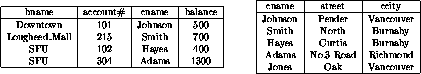Figure 3.1:   The deposit and customer relations.

• It has four attributes.
• For each attribute there is a permitted set of values, called the domain of that attribute.

• E.g. the domain of bname is the set of all branch names.
Let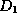denote the domain of bname, and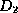,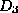and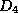the remaining attributes' domains respectively.

Then, any row of deposit consists of a four-tuple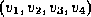where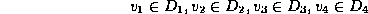In general, deposit contains a subset of the set of all possible rows.

That is, deposit is a subset of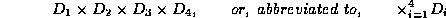In general, a table of n columns must be a subset of2. Mathematicians define a relation to be a subset of a Cartesian product of a list of domains. You can see the correspondence with our tables.

We will use the terms relation and tuple in place of table and row from now on.

3. Some more formalities:
• let the tuple variablerefer to a tuple of the relation.
• We say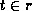to denote that the tupleis in relation.

• Then[bname] == the value ofon the bname attribute.

• So[bname] == ``Downtown'',

• and[cname] == ``Johnson''.
4. We'll also require that the domains of all attributes be indivisible units.
• A domain is atomic if its elements are indivisible units.
• For example, the set of integers is an atomic domain.

• The set of all sets of integers is not.

• Why? Integers do not have subparts, but sets do - the integers comprising them.

• We could consider integers non-atomic if we thought of them as ordered lists of digits.Next: Database Scheme Up: Structure of Relational Previous: Structure of Relational

Page created and maintained by Osmar R. Zaï ane
Last Update: Wed Sep 20 15:45:57 PDT 1995